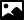## Data Category chemical_formula

### GeneralCategory name
chemical_formula
Required in PDB entries
no
Category group membership
chemical_group
Used in current PDB entries
No

### Category Relationship DiagramsView full category relationship diagram including all dictionary data categories

### Category Description```               Data items in the CHEMICAL_FORMULA category would not, in
general, be used in a macromolecular CIF. See instead the
ENTITY data items.

Data items in the CHEMICAL_FORMULA category specify the
composition and chemical properties of the compound. The formula
data items must agree with those that specify the density,
unit-cell and Z values.

The following rules apply to the construction of the data items
_chemical_formula.analytical, _chemical_formula.structural and
_chemical_formula.sum. For the data item
_chemical_formula.moiety, the formula construction is broken up
into residues or moieties, i.e. groups of atoms that form a
molecular unit or molecular ion. The rules given below apply
within each moiety but different requirements apply to the way
that moieties are connected (see _chemical_formula.moiety).

(1) Only recognized element symbols may be used.

(2) Each element symbol is followed by a 'count' number. A count
of '1' may be omitted.

(3) A space or parenthesis must separate each cluster of (element
symbol + count).

(4) Where a group of elements is enclosed in parentheses, the
multiplier for the group must follow the closing parenthesis.
That is, all element and group multipliers are assumed to be
printed as subscripted numbers. (An exception to this rule
exists for _chemical_formula.moiety formulae where pre- and
post-multipliers are permitted for molecular units.)

(5) Unless the elements are ordered in a manner that corresponds
to their chemical structure, as in
_chemical_formula.structural, the order of the elements within
any group or moiety should be:  C, then H, then the other
elements in alphabetical order of their symbol. This is the
'Hill' system used by Chemical Abstracts. This ordering is
used in _chemical_formula.moiety and _chemical_formula.sum.```

#### Category Example

```
_chemical_formula.entry_id          'TOZ'
_chemical_formula.moiety            'C18 H25 N O3'
_chemical_formula.sum               'C18 H25 N O3'
_chemical_formula.weight            303.40```